AVO Modelling of the South-East Niger Delta, Nigeria | OMICS International
Journal of Remote Sensing & GIS

# AVO Modelling of the South-East Niger Delta, Nigeria

 Uko ED1 and Emudianughe JE2* 1Department of Physics, Rivers State university of Science and technology, PMB 5080, Port Harcourt, Nigeria 2Department of Earth Sciences, Federal University of Petroleum Resources, Effurun, Delta State, Nigeria Corresponding Author : E Emudianughe Department of Earth Sciences Federal University of Petroleum Resources Effurun, Delta State, Nigeria Tel: +2348055501152 E-mail: [email protected] Received August 20, 2014; Accepted September 19, 2014; Published September 23, 2014 Citation: Uko ED, Emudianughe JE (2014) AVO Modelling of the South-East Niger Delta, Nigeria. J Geophys Remote Sensing 3:131. doi:10.4172/2169-0049.1000131 Copyright: © 2014 Uko ED, et al. This is an open-access article distributed under the terms of the Creative Commons Attribution License, which permits unrestricted use, distribution, and reproduction in any medium, provided the original author and source are credited.

Visit for more related articles at Journal of Remote Sensing & GIS

#### Abstract

Amplitude Versus Offset (AVO) modelling was carried out using pre-stack seismic and well-log data in the south-east Coastal Swamp of Niger Delta. The results of the work demonstrated that seismic pres-stack and well-log data provided valuable information in modelling a reservoir and other important intervals in wells. Amplitude-Versus-Angle (AVA)/ Amplitude-Versus-Offset (AVO) extracted from pre-stack seismic data identified gas sand of AVO Class 3 type. Sand- shale lithology is deduced, with sandstone volume decreasing with increasing depth, while shale volume increases with depth. Porosity and permeability showed decreasing trend with depth for both sandstone and shale units. Velocity, GR (API), Poisson’s ratio, V p /V s and density increase with increasing depth. Compressional-velocity V p and shear-wave V s are linearly related, and mudrock line equation is established for the study area: V p =0.807Vs+1.600. The results of this work can be used as an Exploration tool for the identification or evaluation of prospective areas, locations and also for feasibility studies during an appraisal activity.

 Keywords AVO modelling; AVO Gradient; Intercept; Fluid factor; Reservoir; Niger Delta Introduction The theory of “AVO” is based on the idea that changes in seismic reflection amplitude with offset (AVO effects), and these changes are due to contrasts in the elastic or physical properties of rocks . This amplitude contrast is primarily due to the differences in the lithology and fluid content in rocks above and below the reflector . In this study, pre-stack seismic inversion data is integrated with well logs to characterize the reservoir in the Niger Delta Nigeria to extract Amplitude-Versus-Angle (AVO) relations, derive intercept, gradient, fluid factor for identifying oil, gas and brine, and non-hydrocarbon sands, and to predict the petrophysical characteristics of reservoir rock in the area of study. Seismic pres-stack and well-log data provides valuable information in modelling a reservoir and other important intervals in wells. Amplitude-Versus-Angle (AVA)/Amplitude-Versus-Offset (AVO) can be extracted from pre-stack seismic data; the results of the amplitude-angle cross plots can help to identify gas sand of AVO Class 3 type. Sand-shale lithologycan deduced, with sandstone volume decreasing with increasing depth, while shale volume increases with depth. Porosity and permeability decrease with depth for both sandstone and shale units. Velocity, GR (API), Poisson’s ratio, Vp/Vs and density increase with increasing depth. Theoretical Background When seismic waves hit a boundary, part of the energy is reflected while part is transmitted . If the angle of incidence is not zero, P wave energy is partitioned further into reflected and transmitted P and S components (Figure 1). The amplitudes of the reflected and transmitted energy depend on the contrast in physical properties across the boundary, namely compressional wave velocity (Vp), shear wave velocity (Vs), density (ρ) and the angle-of-incidence of the original ray. The angles for incidence, reflected and transmitted rays at a boundary are related according to Snell’s law (Figure 1):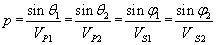(1) where VP1=P-wave velocity in medium 1; VP2=P-wave velocity in medium 2; VS1=S-wave velocity in medium 1; VS2=S-wave velocity in medium 2; θ1=incident P-wave angle, θ2=transmitted P-wave angle, φ1=reflected S-wave angle, φ2=transmitted S-wave angle, and p is the ray parameter. Shuey (1985) equation approximated (Aki et al 2002) to generate the Gradient and Intercept :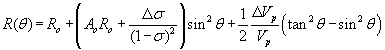(2) where Ro is the normal-incidence P-P reflection coefficient, Ao is given by: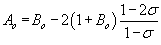(3) and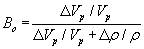(4) where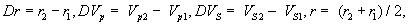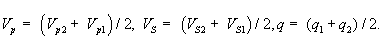The coefficients of Shuey’s approximation form the basis of various weighted stacking procedures. “Weighted stacking”, here also called “Geostack” , is a means of reducing prestack information to AVO attribute traces versus time. We accomplished this by calculating the local angle of incidence for each time sample, then performing regression analysis to solve for the first two or all three coefficients of an equation of the kind: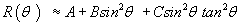(5) where A is the “zero-offset” stack, B is commonly referred to as the AVO “slope” or “gradient”, and the third term becomes significant in the far-offset stack. Aki et al 2002 approximated Zoeppritz equations in terms: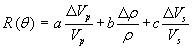(6) where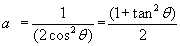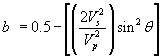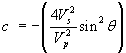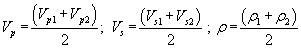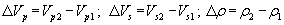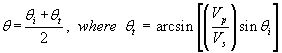Smith and Gidlow (1987) rearranged Equation (6) to give: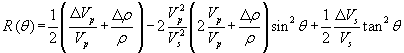(7) Next, they simplified Equation (7) by removing the dependence on density, using Gardner et al. (1974) , Equation: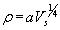(8) which when differentiated gives: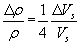(9) Substituting Equation (9) into Equation (7) gives: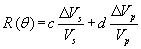(10) where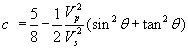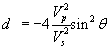Equation (10) solved by least squares to derive weights that can be applied to the seismic gather to produce estimate of both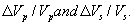. Smith and Gidlow (1987) also derived two other types of weighted stacks, the “pseudo-Poisson’s ratio reflectivity”, defined as: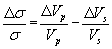(11) and the “fluid factor” stack. To derive the fluid factor, Smith and Gidlow used ARCO mudrock equation, which is the straight-line fit that appears to hold for water-bearing clastics around the world. The equation is written as: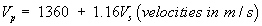(12) Equation (12) when differentiated gives: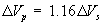(13) Equation (13) expressed in ratio form gives: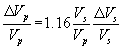(14) Smith and Gidlow (1987) observed that Equation (14) only holds for wet case. In a hydrocarbon reservoir, it does not hold, and “fluid factor” residual is defined as: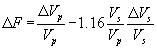(15) In our area of study, Equation (12) was derived to be: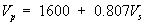Recall approximations to the Zoepprite equations: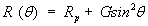(16) where Rp=P-wave intercept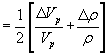G=Gradient =Rp – 2Rs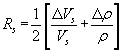In the Smith and Gidlow (1987) approximations, the actual physical parameters,were estimated. However, recalling the Gardner et al. (1974) approximation that was used by Smith and Gidlow (1987) Equation 8, we show that both methods can be equated. First, substitute Equation (9) into the relationship for Rp to get: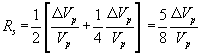(17) which implies: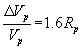(18) Now, substituting Equation (9) into the relationship for Rs we get: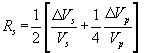(19) Or: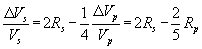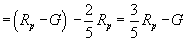(20) Thus,are simply linear combinations of Rp and G. Recall Pseudo-Poisson’s ratio reflectivity in Equation (11):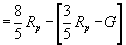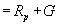(21) Now “Fluid factor” F: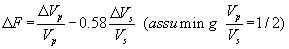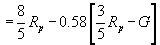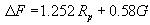(22) Geology of the study area The area of study lies within Coastal Swamp depobelt region of the Niger Delta, between longitudes 7o to 8o E and latitudes 4° to 4.5° N (Figures 2 and 3) . The onshore portion of the Niger Delta Province is delineated by the geology of southern Nigeria and south-western Cameroon. The northern boundary is the Benin flank an east-northeast trending hinge line south of the West Africa basement massif. The north-eastern boundary is defined by outcrops of the Cretaceous on the Abakaliki High and further east-south-east by the Calabar flank - a hinge line bordering the adjacent Precambrian [7,8]. From the Eocene to the present, the delta has prograded south-westward, forming depobelts that represent the most active portion of the delta at each stage of its development . These depobelts form one of the largest regressive deltas in the world with an area of some 300,000 km2 , a sediment volume of 500,000 km3 , and a sediment thickness of over 10 km in the basin depocenter . The Niger Delta Province contains only one identified petroleum system [9,12]. This system is referred to here as the Tertiary Niger Delta (Akata-Agbada) Petroleum System. Petroleum in the Niger Delta is produced from sandstone and unconsolidated sands predominantly in the Agbada Formation (Figure 4). Known reservoir rocks are Eocene to Pliocene in age, and are often stacked, ranging in thickness from less than 15 meters to 10% having greater than 45 meters thickness. The primary source rock is the upper Akata Formation, the marine-shale facies of the delta, with possibly contribution from interbedded marine shale of the lowermost Agbada Formation. Oil is produced from sandstone facies within the Agbada Formation, however, turbidite sand in the upper Akata Formation (Figure 4) is a potential target in deep water offshore and possibly beneath currently producing intervals onshore. A variety of structural trapping elements exists, including those associated with simple rollover structures; clay filled channels, structures with multiple growth faults, structures with antithetic faults, and collapsed crest structures. The primary seal rock in the Niger Delta is the interbedded shale within the Agbada Formation. The shale provides three types of seals-clay smears along faults, interbedded sealing units against which reservoir sands are juxtaposed due to faulting, and vertical seals. Methodology Extraction of various angle of incidence and offsets The synthetics were generated using a program called Land Mark and Hamsel Rossel Workstations which have the capability of generating the synthetics at various offsets angles (Figure 5). For each case, we needed to know the angle of incidence for all gathers, offsets, and time (depth) samples. Given the velocity model and the source receiver location, we determined the angle of incidence using a ray tracing procedure. The next step was to pick all amplitude values (of a given CMP gather) for all offsets for each time sample. Because amplitudes are fundamental in seismic analysis, correct management of amplitudes is very important in reservoir characterization studies [14,15]. Also, as amplitudes are of critical importance in the seismic inversion studies, correct amplitude processing was essential. Then we analyze amplitude as a function of angle of incidence after converting each offset to its corresponding angle using the ray tracing procedure. Pre-stack migration (Figure 6) was used on data for the AVO analysis, because it would collapse the diffractions at the target depth to be smaller than the Fresnel zone and therefore increase the lateral resolution. Pre-stack time migration (PSTM) was preferred to prestack depth migration (PSDM), because the former tends to preserve amplitudes better . An amplitude-preserving PSDM routine was applied since it is the most accurate. Finite-difference approach for the pre-stack migrations was used since it improves the stratigraphic resolution, data quality, and location accuracy of AVO targets [17,18]. Well logs conditioning Bulk density, compressional sonic, gamma ray and resistivity logs from 5 Wells were used for the study. The well log quality was fair over the entire region of interest. Check shot TZ-data (Figure 7) and well markers (Tops and Bases of horizons) were also used for the study. The well logs were carefully conditioned or edited prior to their use in a modelling workflow. This means we have to take great care to correctly treat the log data through shales, across drilling breaks, casing points, and washouts. In all cases the log data were edited, normalized, and interpreted before they were used in a reservoir study. Several specific analysis steps were followed : (i) De-spike and filter to remove or correct anomalous data points. (ii) Normalize logs from all of the selected wells to determine the appropriate ranges and cut-offs for porosity, clay content, water resistivity and Saturation. (iii) Compute the volumetric curves such as total porosity, Vclay, and Sw. (iv) Correct sonic and density logs for mud filtrate invasion if needed. (v) Compute Vshear on all wells. All available logs in the field were validated. Logs were checked and confirmed to be depth-matched. Borehole environmental corrections were applied to all the available logs. Logs with multiple runs were spliced. Determination of lithology and volume of mineralogy: A composite log comprising Gamma-ray log (Figure 8) was used to delineate the lithologies at the pre-determined depth intervals. The American Petroleum Institute (API) values ranges from sandstone line 0 to shale line 125. As the signature of the log move towards the higher values, the formation becomes shalier. The delineation approach enabled us to estimate and establish the lithological sequence of the formation of the study area. To determine the ratio of sand to shale of the subsurface geology of the study area, Gamma ray log run for the different wells were delineated into sections with two litho faces, namely, sandstones and shale. The gamma ray log reflects the shale content of sedimentary formations. Clean sandstones and carbonates normally exhibit a low level of natural radioactivity, while clay minerals and fluid particles in shales show higher levels of radioactivity due to adsorption of the heavy radioactive elements. Basically gamma ray log is useful for location of shales and non-shaly beds and most importantly, for general correlation . Clean sandstones were delineated as with log signatures increasing towards the sand-line that is low API unit ranging between 0 and 20 API units. For sandy-shales it ranges from 20 to 100 API units. While shales have API unit values of 100 and above with log signatures moving towards the shale line showing decrease in rate of sedimentation and overall decrease in energy; identified as fluvial environments and transgress sequences. The amount of each lithofacies was then estimated by counting the interval of a particular lithofacies and then assigns a fraction of this to the total interval within the sand-shale lines which then expressed as a percentage. Checking the quality of the gamma ray log with the response of the density log, it was observed that the gamma ray adequately separates sands from shale as shown in Figure 8. The percentages of sandstones and shales were estimated using Gamma ray logs, Figure 8. The API values indicate sand and shale domains. From the Header of the log, the API values ranges from 0 to 125. As the values increases, the formation lithology becomes shalier. Determination of porosity: It is generally accepted among geoscientists that porosity calculation from bulk density logs is more accurate [21-23]. To calculate the porosity φ, we use the rock matrix density, ρma, the fluid density, ρf, and the bulk density, ρb. The average rock density in the sandstones research reports is 2.66gcm-3. The average rock density in the shales is 2.65gcm-3. The fluid density depends on whether the well encountered water or hydrocarbons. This was determined by the electrical resistivity log. The hydrocarbon density was calculated from composition and phase considerations, oil=0.80 gcm-3. and gas=0.6 gcm-3. The water density used was 1 gcm-3. If Gamma Ray value is below a threshold value, in our case 100 GAPI, the lithology is interpreted to be sandstone, Figure 8. Porosity was determined from the formula.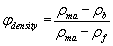(23) where ρma=matrix (or grain) density, ρf=fluid density and ρb=bulk density (as measured by the tool and hence includes porosity and grain density. Determination of sonic velocity: This was based on the fact that, sonic transit time is directly related to the acoustic velocity which is a function of formation lithology and porosity. The sonic log is simply a recording of the time required for a sound wave to traverse one foot of formation known as interval transit time . Sonic log is also a measure of a formation capacity to transmit sound waves. Geologically, this capacity varies with lithologies and rock texture, notably porosity, when the lithology is known . This makes the sonic log very useful as a porosity log. Integrated sonic transit times are also useful in interpreting seismic records. A sudden increase in transit time with depth indicates the presence of abnormal pressure. The sonic transit time values were obtained using the simple ratio method. The Sonic log velocities were crossed- checked with the correlative two-way-travel (TWT) seismic velocity (checkshots) data. The interval transit time values were picked at chosen depth interval. The acoustic velocities were obtained or computed by taking the inverse of the interval transit time Δt.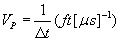(24) Poisson’s ratio, σ, is defined in the relation as: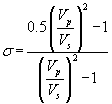(25) Determination of shear and compressional acoustic impedances: The wave propagation depends on the property of the medium called seismic impedance I which is the product of wave velocity (V) and density (ρ) of the rock (I=ρV). If a seismic wave passes through the boundary of two different rocks with varying impedances, a portion of the seismic wave energy reflects, while some transmits through the boundary. The amplitude of the reflected wave called reflection coefficient (R) depends on the impedance contrast between the two layers that created the wave.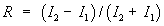(26) where Ii=ρv. From seismic study we have travel time, which is the time it takes for a reflection to arrive at the receiver from a boundary. Shear Acoustic Impedance, Is, is determined as: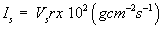(27) where Vs is in ms-1, and ρ is in gcm-3. Compressional Acoustic Impedance, Ip, is determined as: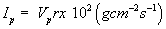(28) where Vp is inms-1,, and ρ is in gcm-3. Determination of shear and bulk moduli of elasticity: From the equation of Shear wave velocity, Shear Modulus of Elasticityμ was determined: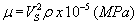(29) where Vs is in ms-1 and ρ is in gcm-3, while Bulk Modulus of Elasticity was computed using the equation: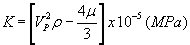(30) Determination of permeability (K): Formation Factor for shaly sands [25-27]: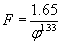(31) Permeability (K) relates to Formation Factor :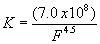(32) Determination of water saturation: To calculate water saturation Sw of un-invaded zone, the method used requires a water resistivity Rw value at formation temperature calculated from the porosity and resistivity logs within clean water zone, using the inverse Archie method: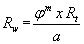(33) where Rw is the water resistivity at formation temperature, φ and Rt are the total porosity and deep resistivity values in the water zone respectively. Tortuosity factor represented as “a” and “m” is the cementation exponent, usually 2 for sands . In the water zone, saturation should be equal to 1, as water resistivity Rw at formation temperature is equal to Rwa . We used m=1.5, a=2, and Rt was read from the Log. The Archie Equation relates water saturation Sw to formation water resistivity as follows: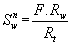(34) where Sw=water saturation; n=saturation exponent=2;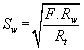(35) Substituting for F from Equation (31), Equation (35) becomes: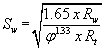Results and Discussion Angle Versus Offset (AVO) Studying the synthetics for AVO meant that a depth measured in time was selected on basis of either it was a shale/gas-sand interface, shale/oil-sand interface or shale/brine-sand interface and amplitudes for the various offset synthetics (hydrocarbon and brine) were extracted at depths of 1900 and 2000ms, which is likely at the point anisotropy start occurring . The fluid curves were used to identify the hydrocarbon or brine interface for each of the synthetics under study. After extracting the amplitudes (Table 1), the amplitudes were crossplotted against angles (Figures 9 and 10) to bring out the relationship between hydrocarbon/brine and amplitude anomaly of the synthetic seismograms. In Figure 9, amplitude decreases with increase with offset (angle) at the depth of 1900ms. Both intercept and gradient are negative having positive product of intercept and gradient. In Figure 10, amplitude decreases with increase of offset having negative intercept and gradient for hydrocarbon, while amplitude gradually increases with increase in offset. The product of the intercept and gradient is negative indicating brine-bearing sands . AVO Class 3 has been observed in the interval 1900 ms and 2000 ms. According to Castagna (1987), the top reservoirs are categorised, based on their amplitude behaviour as a function of offset on a CDP gather when filled with hydrocarbons: - Class 1 Large positive Ro amplitude that stays positive with offset (dimming of reflection on stack). - Class 2 Small positive Ro that is transitioned into negative amplitudes with offset (dimming/brightening of reflection and polarity flip). - Class 3 Negative Ro amplitude that becomes more negative with further offset (brightening of reflection). - Class 4 Negative amplitude becomes less negative with offset (dimming of reflection on stack). AVO Gradient (G), AVO Intercept (Rp) and Fluid Factor, ΔF By using Equations 16, 18, 20 and 22, AVO gradient and intercept and fluid factor were computed and plotted, observed that ΔF=0 for wet reservoirs, and nonzero for gas-filled reservoirs. In this study, F displayed in Figures 11, 12 and 14 is nonzero and therefore is indicative of a gas reservoir. Notice that the AVO gradient, AVO intercept and Fluid factor are negatively-correlated (Figures 11 and 13). The classification of the AVO response is based on position of the reflection of interest on the Gradient versus Intercept cross-plot (Figure 11), and in this case it is in the IV quadrant, and therefore it is Class 1 gas sand as confirmed in Figures 9 and 10. Petrophysical properties Well AZU1 a representative of the Wells which penetrated the depth of interest was used to compute the reservoir Petrophysical properties, which are cross-plotted and are presented in Figure 15-29. Figure 9 shows the composite log sheet. To the right end of this figure are displayed side-by-side synthetic and real seismic traces in time (ms) with crest and troughs. The signatures of the logs and the seismic traces reveal that the reservoir is located between depth 1864 m and 2050 m. Moreover, the data from gamma-ray, density and the P-wave logs show that depth 1864 m to 1930 m is gas-sand, highly porous saturated zone, which is indicative of the fluid accumulation zone of the reservoir. The shaley-sand portion of the reservoir falls within the depths 1930 and 2050 m as shown Figure 15 sand/shale-depth cross-plot and Figure 16 the plot of Gamma-ray API values against depth. This zone with the circle indicates a porous sandy formation in the depth region of 1864 and 1930, while shaley-sand formation is at 1930-2050 m. Figure 17 shows density-depth cross-plot while Figure 18 is a porositydepth cross-plot. The porosity plot against depth of Figure 18 shows a decrease in porosity with increasing depth but with a trend deviation in region of the reservoir from 1864 m to 2050 m. Figure 19 is a permeability-depth cross-plot. There is a normal linear decrease of permeability with an increase in depth, but within the reservoir (1864-2050 m) there is an increase of permeability with an increase in depth (Figure 19) as shown in the circled part of the depth-permeability cross-plot. This indicates a highly permeability zone with values ranging between 282.878 and 15,987.853 md. When permeability is cross-plotted against porosity, there is an exponential increase of permeability with porosity (Figure 20) showing that the reservoir is highly porous. There is a linear decrease of water saturation with an increase in depth, but within the reservoir (1864-2050 m) there is an increase of water saturation with an increase in depth (Figure 21) as shown in the circled part of the depth-water saturation cross-plot. Water saturation ranges between 0.761 and 0.806. This indicates a highly water-saturated zone. When water saturation is cross-plotted against permeability, there is an exponential increase of permeability with water saturation (Figure 22) showing that the reservoir is highly permeable. There is a linear relationship between water saturation and porosity (Figure 23) showing that the reservoir is highly porous and permeable. Figure 21 is water saturation-depth cross-plot; Figure 22 is permeability-water saturation cross-plot, while Figure 23 is porosity-water saturation cross-plots. From the signatures of these profiles, the reservoir is highly porous, permeable with high water saturation. Figure 24 displaying Vp-depth cross-plot shows the linear variation of compressional wave velocity Vp with depth. Figure 25 is a cross-plot of Vp versus Vs. The plot of P-wave velocity against S-wave velocity shows an almost linear trend as presented by Figure 25. This Figure 25 is a cross-plot of Vp against shear wave velocity Vs for the sand and shaley sand sequences. The probable hydrocarbon-charged zone has Vp ranging from 2110 to 2570 m/s and Vs from 873 to 1091 m/s. This work shows that there is a linear relationship between P-wave velocity and S-wave velocity for brine saturation. In the Gulf of Mexico, Castagna et al. established the Mudrock Line to be Vp=1.16Vs+1.36 for velocity in km/s. From Figure 23 Mudrock Line for the study area is here established to be: Vp=0.807Vs+1.600 (36) where velocity is in km/s. Generally, the Mudrock Line is referred to as being: Vp=A*Vs+B(37) where A=0.807 and B=1.600 are constants specific to the study area in Niger Delta. In the absence of shear-wave data, Equation (36) can be used to estimate the shear-wave velocity when P-wave velocity is available. Figure 26 is Poisson’s ratio-Vp cross-plot while Figure 24 is Vp/Vs- Vp cross-plot. Mudrock Line is also plotted using Poisson’s ratio versus P-wave velocity and Vp/Vs ratio versus P-wave velocity Vp as shown in Figure 26 and 27 respectively. Notice that the plot of Poisson’s ratio against P-wave velocity (Figure 26) shows that the lowest Poisson’s ratio is 0.1. On the Vp/Vs ratio against P-wave velocity plot (Figure 27), the curve approaches 1.5 asymptotically. These values (σ=0.1 and Vp/Vs=1.5) represent the “dry rock” value for a dry porous sandstone. Thus, the “mudrock line approaches the “dry rock” line as P-wave velocity increases. Figure 28 shows Vp/ Vs-depth cross-plot showing the plot of both S-wave velocity and P-wave velocities against depth. This profile shows that P-wave velocity is higher than S-wave velocity, but all in the increasing trend throughout the investigated depth, but an anomaly of the velocity trend was observed at depth range 1864 m to 2050 m indicated by the circle, which corresponds to the reservoir location in the subsurface as shown by Figure 28. The Vp/ Vs ratio for the depth of investigation ranges from 2.17 to 3.18. the abnormally low Vp/ Vs ratio values less than 3.00 within the depth correspond to some of the sand lithology identified from the Gamma-ray log. This zone is indicated as probable hydrocarbon zone where Vp/ Vs ration ranges from 2.35 to 3.18. these values are consistent with the observations of Gardner and Harris (1968) , Gregory (1977), Hamilton (1971), and Tatham (1982) [32-35]. The depth ranges between 1864 and 1933. Figure 29 is a cross-plot of Poisson’s ratio versus depth, showing the variation of Poisson’s ratio with depth for the depth under investigation. It shows a decrease of in Poisson’s ratio with depth. The abnormally low Poisson’s ratio (kick) values (less than 0.40) at specific depth intervals correspond to some of the sand lithologies identified from Gamma-ray log. These low Poisson’s ratio are indicated as the probable hydrocarbon zone. Poisson’s ratio ranges from 0.365 to 0.390 for the depth ranges between 1864 and 1930. Compressional-velocity Vp and shear-wave Vs are linearly related, and mudrock line equation is established for the study area: Vp=0.807 Vs+1.600. Conclusion The signatures of the logs and the seismic traces reveal that the reservoir is located between depth 1864 m and 2050 m. The data from gamma-ray, density and the P-wave logs show that depth 1864 m to 1930 m is gas-sand, highly porous saturated zone, which is indicative of the fluid accumulation zone of the reservoir. The shaley-sand portion of the reservoir falls within the depths 1930 and 2050 m. P-wave velocity is higher than S-wave velocity, but they are all in the increasing trend throughout the investigated depth, but an anomaly of the velocity trend was observed at depth range 1864 m to 2050m. This corresponds to the reservoir location in the subsurface. References

#### Tables and Figures at a glanceTable 1

#### Figures at a glance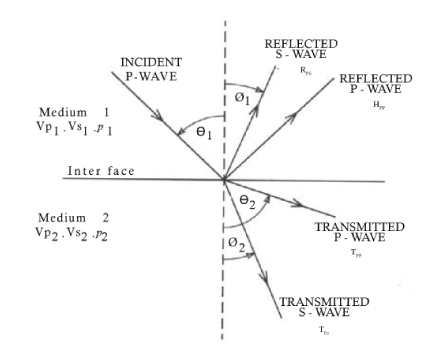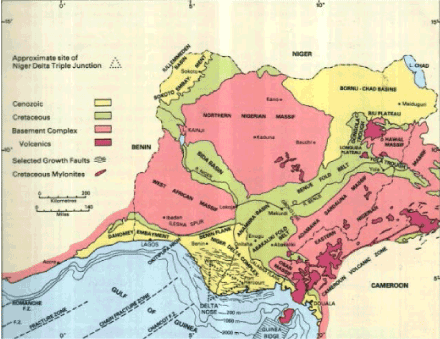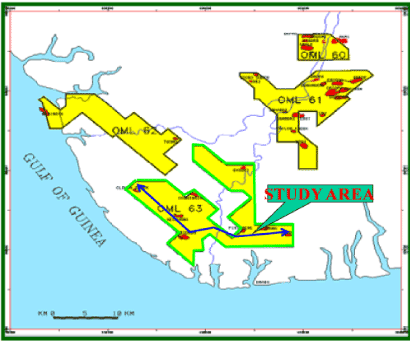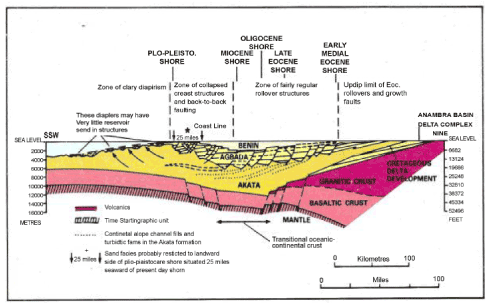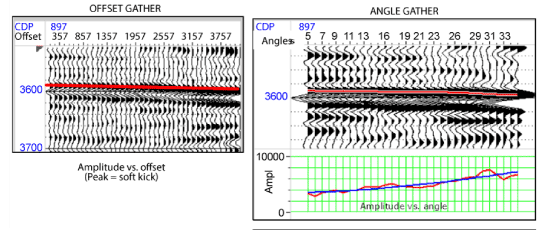Figure 1 Figure 2 Figure 3 Figure 4 Figure 5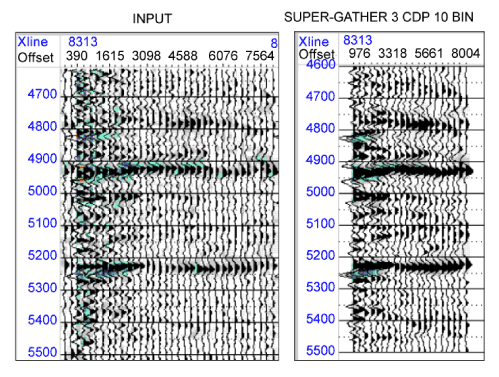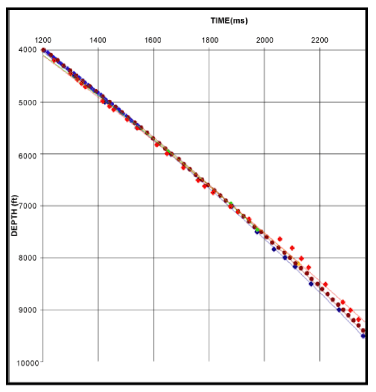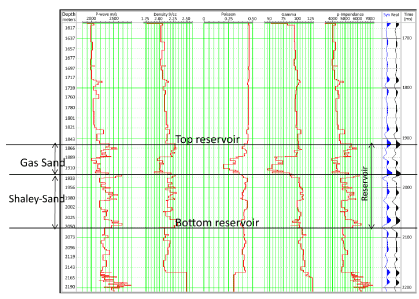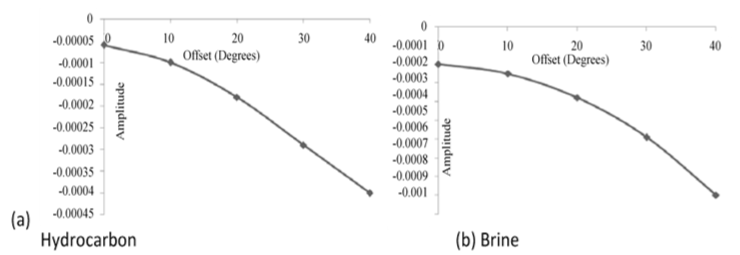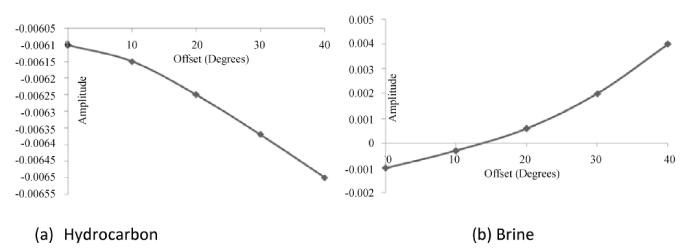Figure 6 Figure 7 Figure 8 Figure 9 Figure 10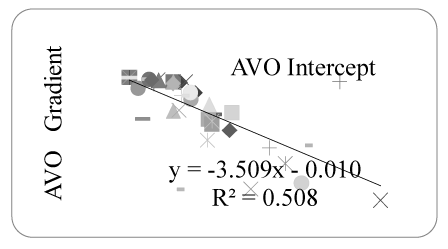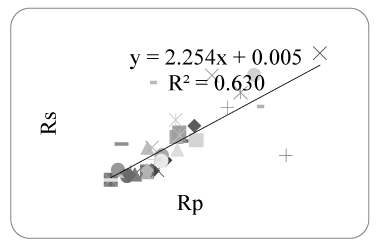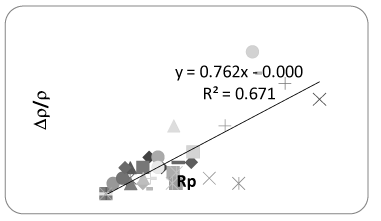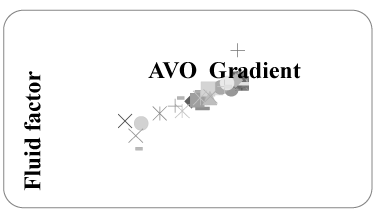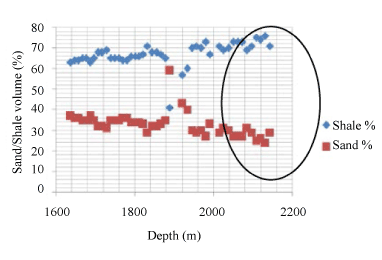Figure 11 Figure 12 Figure 13 Figure 14 Figure 15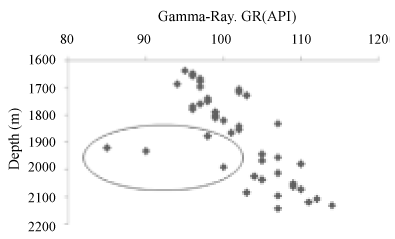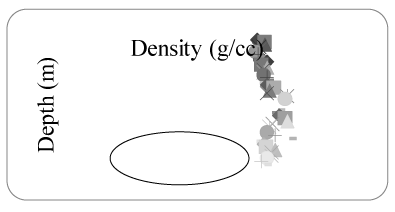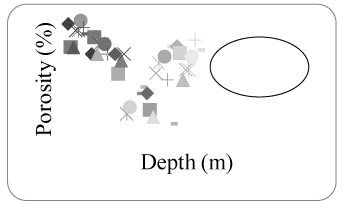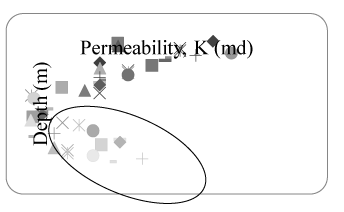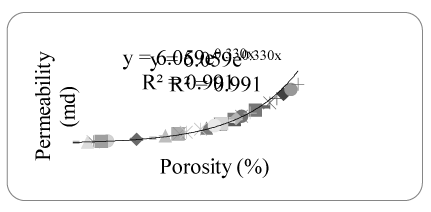Figure 16 Figure 17 Figure 18 Figure 19 Figure 20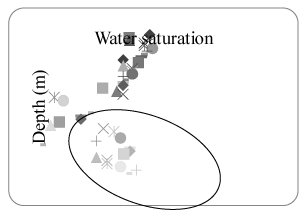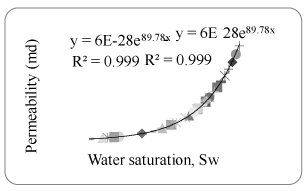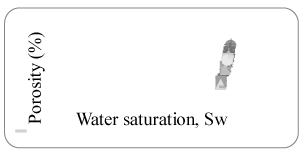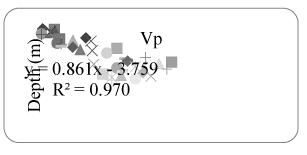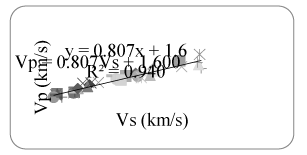Figure 21 Figure 22 Figure 23 Figure 24 Figure 25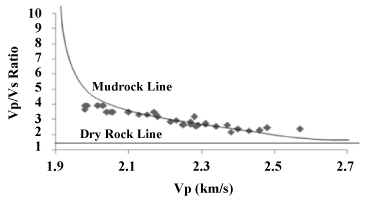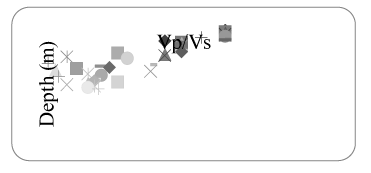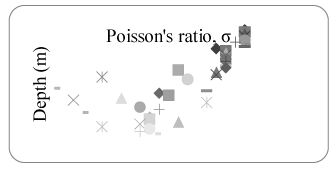Figure 26 Figure 27 Figure 28 Figure 29
Select your language of interest to view the total content in your interested language

### Article Usage

• Total views: 13088
• [From(publication date):
December-2014 - Dec 15, 2019]
• Breakdown by view type
• HTML page views : 9122Can't read the image? click here to refresh